# Understanding how image size is handled in fast.ai

I’m playing with the dog-breed data. I am not clear how size of images is handled by the fast.ai/pytorch library.

The images are generally rectangular 443 wide X 387 high.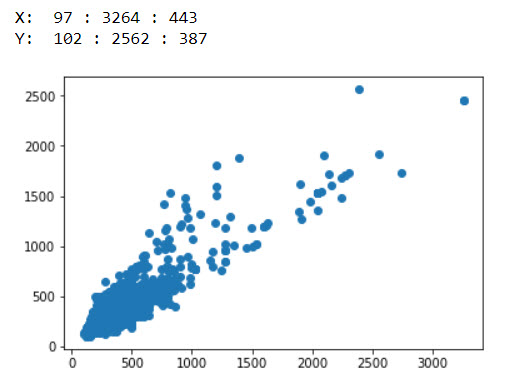The lecture said that ImageNet’s network was trained on pictures either 224x224 or 299x299.
So the average dog-breed picture is bigger than that, and not square.

Stealing boilerplate code from the teacher, I have:

``````sz=224

arch=resnext101_64  #epoch accuracy = 0.9257   (his first run was 83.6%)

tfms = tfms_from_model(arch, sz, aug_tfms=transforms_side_on, max_zoom=1.1)

n = len(list(open(f'{PATH}labels.csv')))-1
val_idxs= get_cv_idxs(n)  #get cross validation indices  [should happen by default according to source]

data = ImageClassifierData.from_csv(PATH, folder='train', csv_fname=f'{PATH}labels.csv', tfms=tfms,
suffix='.jpg', val_idxs=val_idxs, bs=32)  #reduced bs from 64
``````

So what is going to happen? is the `tfms_from_model` going to take every image, chop it square, and resize (up if it’s tiny, or down if it’s big) to `sz=224` pixels square? Then `data` object will be entirely full of 224x224 pictures?

But I’m further-confused by the `get_data(sz, bs)` function the professor showed during his second lecture:

I call `get_data()` with the size and batch-size I need. So I cannot understand what the conditional return does: If the sz parameter >= 300, then leave the `data` object (which was set up with a `tfms` of sz > 300) alone and return it. But if, for some reason, I specifed a sz less than 300, apply `.resize()` directly to the data object and make it bigger ?340x340?

``````Init signature: ImageClassifierData(path, datasets, bs, num_workers, classes)
Docstring:      <no docstring>
File:           ~/fastai/courses/dl1/fastai/dataset.py
Type:           type
``````

Unfortunately there is no docstring for `ImageClassifierData`, so I don’t know what that function is trying to do, or how it is different/overrides whatever `tfms` is doing.

I would appreciate if someone can explain how image size flows through the system and is handled.

4 Likes

@karavshin I got exactly the same question. Did you succeed to understand that?

OK, I think I figured this out.
Actually when the images are loaded to the `data` object - they are cropped to square (side of the square = smaller dimension of original image) and they are resized till the value of `sz` (i.e. 64x64 if `sz=64`).

To make it visual - here is the result of my experiments with couple of them:
Original images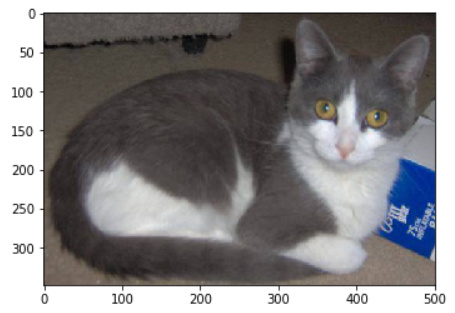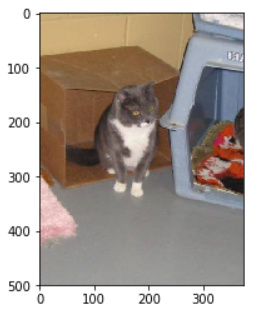`sz = 64`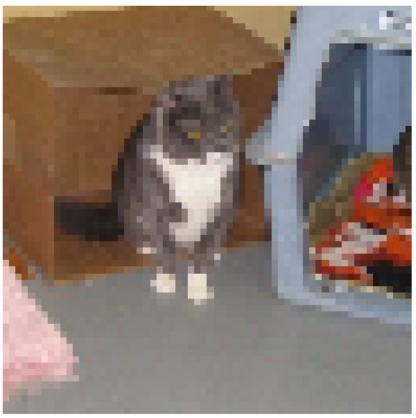`sz=128``sz=32`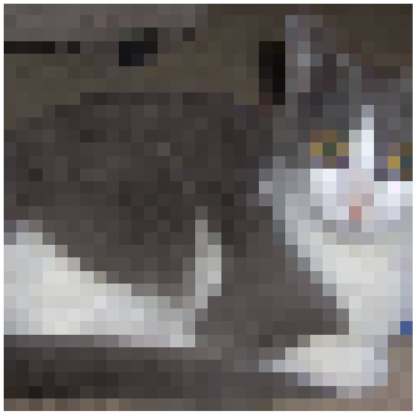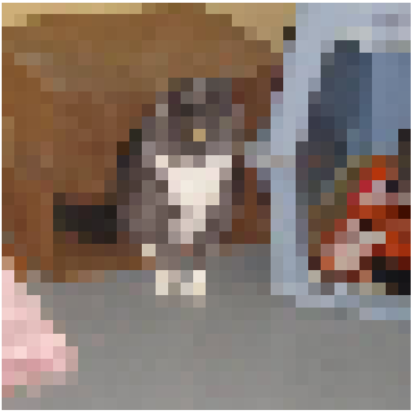You can check out this small notebook for more details.

4 Likes

@t0tem As rectangular image gets cropped, do you think squaring image (without cropping, maybe stretching along smaller side) will improve accuracy. As squaring doesnt lose any data

it will help.My suggestion is to try out each augmentation on mini batch and then combine all and then see the results

Still don’t get the magic of numbers 300 and 340 (if the image is less than 300, then resize to 340)

3 Likes